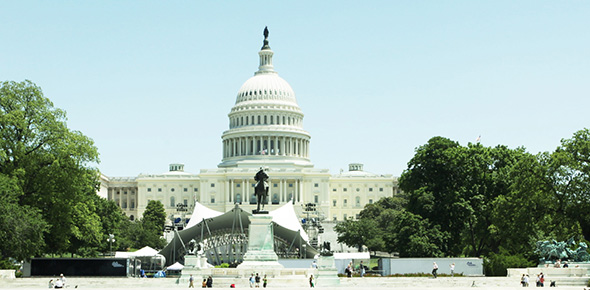# MCQ On Cost Of Capital

5 Questions | Attempts: 1083
ShareSettings.

• 1.
During planning period, marginal cost to raise a new debt is classified as
• A.

Debt cost

• B.

Relevant cost

• C.

Borrowing cost

• D.

Embedded cost

• 2.
Cost of common stock is 14% and bond risk premium is 9% then bond yield is
• A.

1.56%

• B.

5%

• C.

23%

• D.

64.28%

• 3.
In weighted average cost of capital, company can affect its capital cost through
• A.

Policy of capital structure

• B.

Policy of dividends

• C.

Policy of investment

• D.

All of above

• 4.
Risk associated with project and way considered by well diversified stockholder is classified as
• A.

Expected risk

• B.

Beta risk

• C.

Industry risk

• D.

Returning risk

• 5.
Cost of common stock is 13% and bond risk premium is 5% then bond yield is
• A.

\$18

• B.

2.60%

• C.

8%

• D.

18%

## Related TopicsBack to top
×

Wait!
Here's an interesting quiz for you.